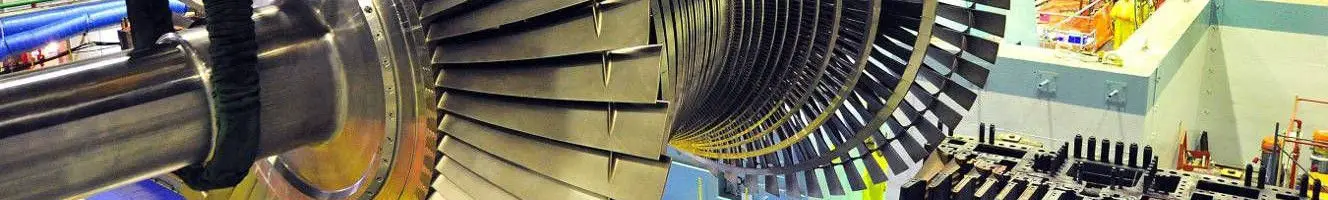# Nuclear EnergyNuclear Power Plant Isar, GermanySpent nuclear fuel poolTurbine of a nuclear plant

# Potential

## Potential energyIn physics, the potential energy is the energy that an object possesses due to its position in a force field or that a system has due to the configuration of its parts.

There are many types of potential energy, but the most common are:

• Gravitational potential energy that depends on the vertical position and the mass of an object.
• Elastic potential energy of an extended spring
• Electric potential energy of a charge in an electric field.

The unit of measurement of the International System of Units for energy is the joule (J).

Potential…

+ info

## Electric powerWe define electricity as the form of energy that results from the existence of a potential difference between two points. When these two points are put in contact by means of an electrical conductor we obtain an electric current.

In physics, the electric potential energy, also called electrostatic potential energy, is the potential energy of the electrostatic field. This is an energy that has an electrical charge distribution, and is linked to the force exerted by the field generated by the distribution itself. Together with magnetic energy, the electric potential energy constitutes…

+ info

## Mechanical energyMechanical energy can be defined as the ability to produce a mechanical work that a body possesses due to causes of mechanical origin, such as its position or its speed. There are two forms of mechanical energy that are kinetic energy and potential energy.

The mechanical energy of a body is the sum of its kinetic energy and its potential energy. Mechanical energy can be partially transformed into other types of energy, such as electrical energy (in a ventilator), and can be obtained by transforming other energies, such as chemical energy (in a person who walks).

The kinetic energy…

+ info

## Chemical energyChemical energy is the potential of a chemical substance to undergo a transformation through a chemical reaction or to transform itself into other chemical substances. Forming or breaking chemical bonds involves energy. This energy can be absorbed or evolved from a chemical system.

The energy that can be released (or absorbed) by a reaction between a set of chemical substances is equal to the difference between the amount of energy of the products and the reactants. This change in energy is called the internal energy of a chemical reaction.

Since the strength of…

+ info

## IonIn physics and chemistry, an ion is an atom or molecule that does not have a neutral electrical charge. A cation with a positive electric charge is called a cation, and an ion with a negative electric charge is anion.

The process of winning or losing electrons (with respect to the neutral atom or molecule) is called ionization. Cations and anions are usually represented by the symbol of the corresponding atom and the symbol "+" or "-", respectively. If the number of electrons gained or lost is greater than one, this is also indicated.

The cations and anions are…

+ info

## Definition of energyAbility of a physical system to produce a job.

This definition, although it is the most widespread, can be misleading because of the vagueness of the term "capacity", and therefore, it is better to define the energy as what, when a work occurs, decreases in an amount equal to the work produced. Energy, then, is measured in the same units as labor.

History of the study of energy

The notion of energy appears for the first time from the investigations of Joule and Carnot on the conversion of heat in mechanical work and thanks to the theory of Helmholtz in which it connects…

+ info

## Kinetic energyKinetic energy is the energy contained in a body due to being in motion. The kinetic energy is the amount of work needed to accelerate a body of a given mass to a certain speed (linear or rotational). This energy obtained during acceleration will remain unchanged as long as this body does not change its speed.

In classical mechanics, the linear kinetic energy (without rotation) of an object of mass m traveling at velocity v is ½ · m · v2. In relativistic mechanics it is a good approximation only when the speed is much less than the speed of light.

+ info